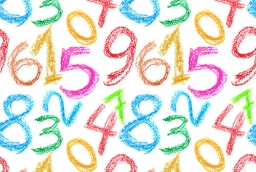# Consecutive 18083

The sum of five consecutive even numbers is 40. Find these numbers.

a =  4
b =  6
c =  8
d =  10
e =  12

### Step-by-step explanation:

a+(a+2)+(a+4)+(a+6)+(a+8) = 40

5a = 20

a = 20/5 = 4

a = 4

Our simple equation calculator calculates it.Did you find an error or inaccuracy? Feel free to write us. Thank you!

Tips for related online calculators
Do you have a linear equation or system of equations and looking for its solution? Or do you have a quadratic equation?